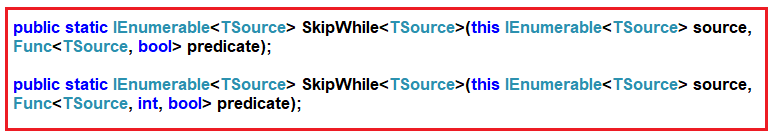# LINQ SkipWhile Method in C#

## LINQ SkipWhile Method in C# with Examples

In this article, I am going to discuss LINQ SkipWhile Method in C# with Examples. Please read our previous article where we discussed the LINQ Skip Method in C# with Examples. As part of this article, we are going to discuss the following pointers which are related to the LINQ Skip Method.

1. What the SkipWhile Method is and its need in C#.NET?
2. Examples of SkipWhille Method using both Method and Query Syntax.
3. Example using Index.
##### LINQ SkipWhile Method in C#

The LINQ SkipWhile Method in C# is used to skip all the elements from a data source or a sequence or a collection until a specified condition is true. Once the condition is failed, then returns the remaining element from the sequence as output. The most important point that you need to remember is, once the condition is failed then the SkipWhile method will not check the rest of the elements even though the condition is true for some of the remaining elements. If this is not clear at the moment, then don’t worry, once we discussed the examples, then you will definitely understand this concept.

The point that you need to remember is that, like the Skip Method, the LINQ SkipWhile Method will not make any changes to the positions of the elements in the data source. There are two overloaded versions available for this SkipWhile Method in C#. They are as shown in the below image.The First version of the LINQ SkipWhile method skips or bypasses the elements from a sequence as long as the specified condition is true. Once the condition failed, it will return the remaining elements from the data source.

The second overloaded version of the SkipWhile method bypass or skips the elements from a sequence as long as the given condition is true and then returns the remaining elements. The second parameter of the function represents the index of the source elements. The element’s index can be used in the logic of the predicate function. If this is not clear at the moment then don’t worry we will discuss this with an example.

##### Example to Understand LINQ SkipWhile Method in C#:

Let us see an Example to Understand LINQ SkipWhile Method in C# using both Method syntax and Query Syntax. In the following example, we created a data source that contains numbers from 1 to 10. Then we skip the elements from the data source using the SkipWhile method with the condition that the number is less than 5. The point that you need to remember is, there is no such operator called skip while available in LINQ to write the query syntax, So, here we need to use the mixed syntax which is also shown in the below example.

```using System;
using System.Collections.Generic;
using System.Linq;
namespace LINQSkipWhileDemo
{
class Program
{
static void Main(string[] args)
{
//Data Source
List<int> numbers = new List<int>() { 1, 2, 3, 4, 5, 6, 7, 8, 9, 10 };

//Skip Numbers which are less than 5 using SkipWhile Method
//Using Method Syntax
List<int> ResultMS = numbers.SkipWhile(num => num < 5).ToList();

//Using Query Syntax
List<int> ResultQS = (from num in numbers
select num).SkipWhile(num => num < 5).ToList();

//Accessing the Result using Foreach Loop
foreach (var num in ResultMS)
{
Console.Write(\$"{num} ");
}

}
}
}```

Output: 5 6 7 8 9 10

##### Example:

Let us modify the position of the elements in the collection. Here we are moving elements 2 and 3 to the end of the collection.

```using System;
using System.Collections.Generic;
using System.Linq;

namespace LINQDemo
{
class Program
{
static void Main(string[] args)
{
List<int> numbers = new List<int>() { 1, 4, 5, 6, 7, 8, 9, 10, 2, 3 };

List<int> ResultMS = numbers.SkipWhile(num => num < 5).ToList();

foreach (var num in ResultMS)
{
Console.Write(\$"{num} ");
}

}
}
}
```

Output: 5 6 7 8 9 10 2 3

As you can see we are getting 2 and 3 in the output even if they are smaller than 5. This is because the SkipWhile method checks the conditions from the beginning of the data source. As long as the condition is true it bypasses the data. So, in our example, for the first two elements, the condition is true so it skips the first two elements. When it checks the third element the condition becomes false. So from that point, it will not check the rest of the elements in the data source even though some of the elements (i.e. 2 and 3 are present at the end of the collections) satisfy the condition.

##### Example:

In the following example, we have a collection of names. Here, we need to skip the names’ starting from the beginning whose length is less than 4.

```using System;
using System.Collections.Generic;
using System.Linq;

namespace LINQDemo
{
class Program
{
static void Main(string[] args)
{
List<string> names = new List<string>() { "Pam", "Rahul", "Kim", "Sara", "Priyanka" };

List<string> namesResult = names.SkipWhile(name => name.Length < 4).ToList();

foreach (var name in namesResult)
{
Console.Write(\$"{ name} ");
}

}
}
}
```

Output: Rahul Kim Sara Priyanka

##### Example: Using Index

Let us use the other overloaded version of the SkipWhile method which takes the index position of the element as a parameter. Here, in the following example, we will check the length of the name should be greater than its index position.

```using System;
using System.Collections.Generic;
using System.Linq;

namespace LINQDemo
{
class Program
{
static void Main(string[] args)
{
List<string> names = new List<string>() { "Sara", "Rahul", "John", "Pam", "Priyanka" };

List<string> namesResult = names.SkipWhile((name, index) => name.Length > index).ToList();

foreach (var name in namesResult)
{
Console.Write(\$"{ name} ");
}